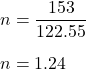## How many moles are in 153’pp grams of KClO3

Question

How many moles are in 153’pp grams of KClO3

in progress 0
7 months 2021-07-13T20:34:39+00:00 1 Answers 8 views 0

n = 1.24 moles

Explanation:

Given that,

Mass = 153 grams

Molar mass of KClO₃ = 122.55 g/mol

We need to find the number of moles.

We know that,

No. of moles = given mass/molar mass

So,So, there are 1.24 moles in 153 g of KClO₃.